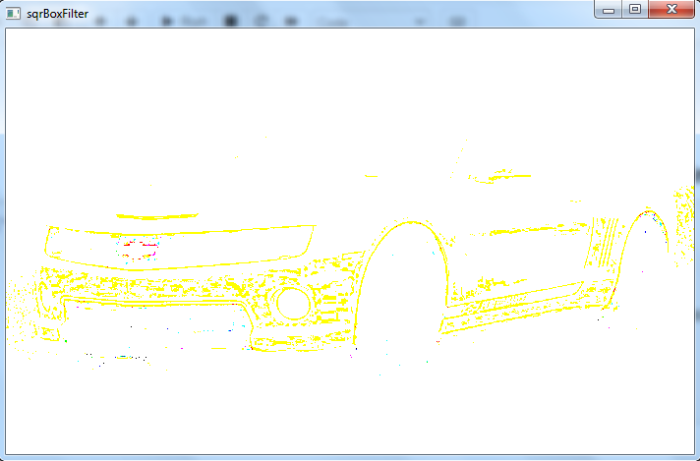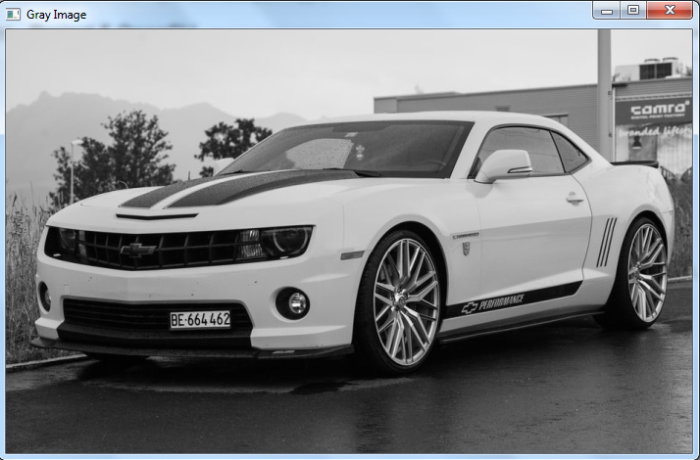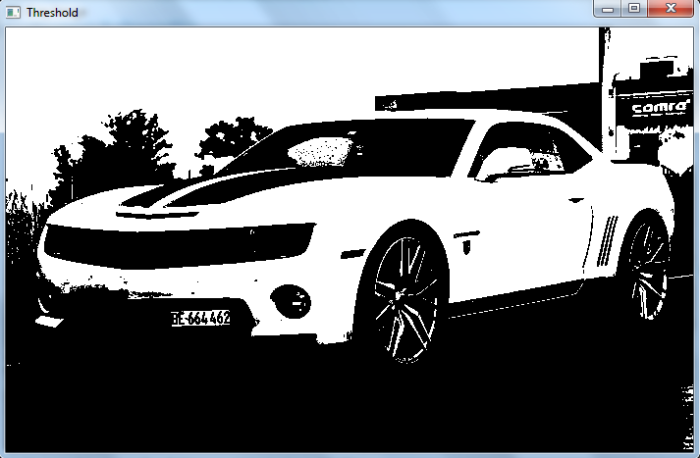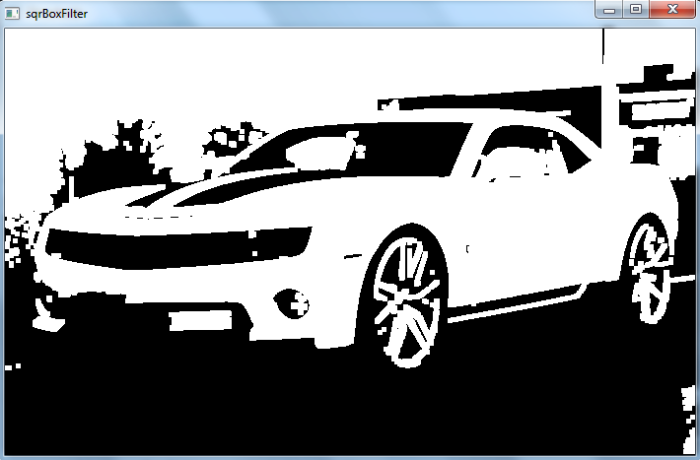# OpenCV Python â€“ How to perform SQRBox filter operation on an image?

We can perform SQRBox Filter operation on an image using cv2.sqrBoxFilter(). It calculates the normalized sum of squares of the pixel values overlapping the filter. We use the following syntax for this method âˆ’

cv2.sqrBoxFilter(img, ddepth, ksize, borderType)


where, img is the input image, ddepth is the output image depth, ksize is kernel size and borderType is border mode used to extrapolate pixels outside of the image.

## Steps

To perform SQRBox filter operation, you could follow the steps given below-

• Import the required library. In all the following examples, the required Python library is OpenCV. Make sure you have already installed it.

• Read the input image using cv2.imread() method. Specify full path of image with the image type (i.e. png or jpg)

• Apply cv2.sqrBoxFilter() filtering on the input image. We pass ddepth, ksize, borderType to the function. We can adjust the ksize for better results.

sqrbox = cv2.sqrBoxFilter(img, cv2.CV_32F, ksize=(1,1), borderType = cv2.BORDER_REPLICATE)

• Display the sqrBoxFilter filtered image.

We will use this image as the Input File in the following examples âˆ’## Example

In this Python program, we apply the SQRBox filter on the color input image with kernel size 1x1.

# import required libraries
import cv2

# apply sqrBoxFilter on the input image
sqrbox = cv2.sqrBoxFilter(img, cv2.CV_32F, ksize=(1,1),
borderType = cv2.BORDER_REPLICATE)
print("We applied sqrBoxFilter with ksize=(1,1)")

# Save the output
cv2.imshow('sqrBoxFilter', sqrbox)
cv2.waitKey(0)
cv2.destroyAllWindows()


## Output

On execution, it will produce the following output âˆ’

We applied sqrBoxFilter with ksize=(1,1)


And we get the below window showing the output âˆ’## Example

In this Python program, we apply the SQRBox filter on a binary image with kernel size 5Ã—5.

# import required libraries
import cv2

gray = cv2.cvtColor(img, cv2.COLOR_BGR2GRAY)
_, thresh = cv2.threshold(gray, 150, 255,cv2.THRESH_BINARY)

# apply sqrBoxFilter on the input image
sqrbox = cv2.sqrBoxFilter(thresh, cv2.CV_32F, ksize=(5,5),
borderType = cv2.BORDER_REPLICATE)
print("We applied sqrBoxFilter with ksize=(5,5)")

# Display the outputs
cv2.imshow('Gray Image', gray)
cv2.waitKey(0)
cv2.imshow('Threshold', thresh)
cv2.waitKey(0)
cv2.imshow('sqrBoxFilter', sqrbox)
cv2.waitKey(0)
cv2.destroyAllWindows()


## Output

On execution, it will produce the following output âˆ’

We applied sqrBoxFilter with ksize=(5,5)


And we get the following three windows showing the outputs âˆ’